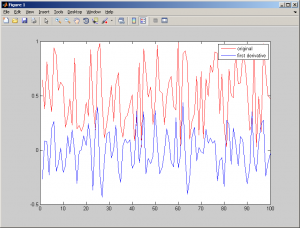# C/C++ First Derivative Code

In calculus, the first derivative test uses the first derivative of a function to determine whether a given critical point of a function is a local maximum, a local minimum, or neither. The idea behind the first derivative test is to examine the monotonicity properties of a function just to the left and right of a given point in its domain. If the function “switches” from increasing to decreasing at the point, then close to that point, it will achieve a highest value at that point. Similarly, if the function “switches” from decreasing to increasing at the point, then close to that point, it will achieve a least value at that point. If the function fails to “switch”, and remains increasing or remains decreasing, then no highest or least value is achieved.

From this first derivative description, I create a C/C++ first derivative code.

```void firstDerivative(float *data, float *firstderv, int nx)
{
int i;
float tmp1, tmp2;

//compute derivative first index
firstderv = data-data;

for(i=1; i<nx-2; i++)  //compute first derivative
{
tmp1 = data[i]-data[i-1];
tmp2 = data[i+1]-data[i];
firstderv[i] = (tmp2+tmp1)/2;
}

//compute derivative last index
firstderv[nx-1] = data[nx-1]-data[nx-2];
}```

Output from this first derivative function is firstderv. firstderv have same size with input data (variable data). This is output from data processed with first derivative code :Source :

http://en.wikipedia.org/wiki/First_derivative_test
1.•2.•3.4.